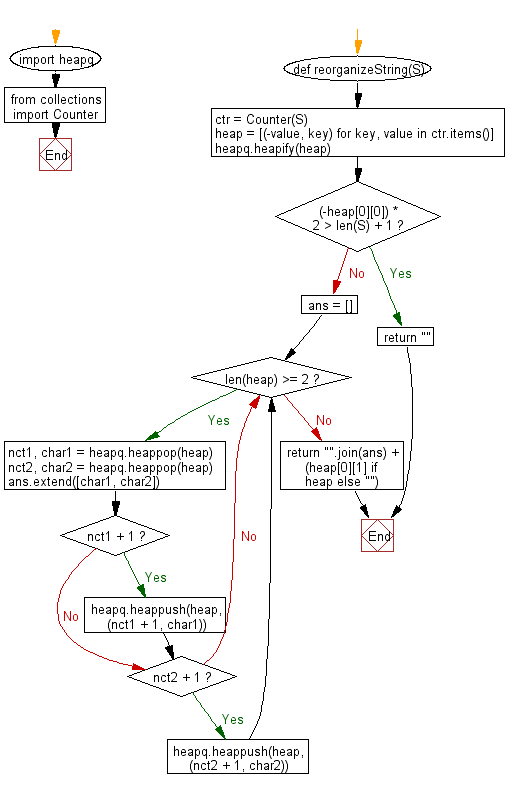﻿ Python heap queue algorithm: Check if the letters of a given string can be rearranged so that two characters that are adjacent to each other are different - w3resource

# Python: Check if the letters of a given string can be rearranged so that two characters that are adjacent to each other are different

## Python heap queue algorithm: Exercise-15 with Solution

Write a Python program to check if the letters in a given string can be rearranged. This is to make sure that two characters that are adjacent to each other are different using the heap queue algorithm.

Note:
If there is no output return the empty string.

Sample Solution:

Python Code:

``````import heapq
from collections import Counter
def reorganizeString(S):
ctr = Counter(S)
heap = [(-value, key) for key, value in ctr.items()]
heapq.heapify(heap)
if (-heap) * 2 > len(S) + 1:
return ""
ans = []
while len(heap) >= 2:
nct1, char1 = heapq.heappop(heap)
nct2, char2 = heapq.heappop(heap)
ans.extend([char1, char2])
if nct1 + 1: heapq.heappush(heap, (nct1 + 1, char1))
if nct2 + 1: heapq.heappush(heap, (nct2 + 1, char2))
return "".join(ans) + (heap if heap else "")

print(reorganizeString("aab"))
print(reorganizeString("abc"))
print(reorganizeString("aabb"))
print(reorganizeString("abccdd"))
```
```

Sample Output:

```aba
abc
abab
cdabcd
```

Flowchart:Python Code Editor:

Have another way to solve this solution? Contribute your code (and comments) through Disqus.

What is the difficulty level of this exercise?

Test your Programming skills with w3resource's quiz.

﻿